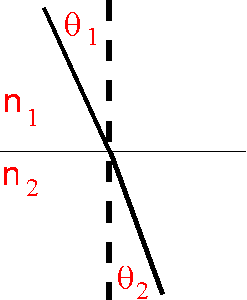### Snell's LawThis form of Snell's law was actually published by Descartes as the law of Sines. Snell did discover the relationship but articulated it in a different way. Today it is the form used by Descartes that is called Snell's law.

The law is defined as:

n1 sinQ1 = n2 sinQ2

Where n is the refractive index and Q the corresponding angles as shown.

The refractive index is the ratio of the speed of light in a vacuum to the speed of light in a given medium.

So, if the top part of the diagram is air, n1 is the speed of light in air and if the bottom part is glass, n2 is the speed of light in glass, both relative to the speed of light in a vacuum.

Snell and Descartes realized that when light went from one medium to another, the angles & refractive indexes of the media determined the path that light took. The relationship is a function of the sine of the angles.

Refraction
Visual Stimulus